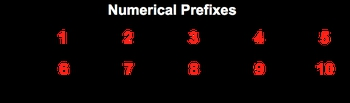Start typing, then use the up and down arrows to select an option from the list.## General Chemistry

Learn the toughest concepts covered in Chemistry with step-by-step video tutorials and practice problems by world-class tutors

3. Chemical Reactions

# Naming Molecular Compounds

Molecular Compounds, also known as covalent compounds, contain only non-metals bonded together.

Naming Molecular Compounds
1
concept

## Naming Molecular Compounds2m
Play a video:
here, we're gonna learn how to name molecular compounds. First off, molecular compounds are the same thing as Covalin compounds. And remember that CO violent compounds are compounds containing Onley. Non metals bonded together. We're gonna say here that they use numerical prefixes. No numerical prefixes are always required because these compounds can combine in different proportions. And our numerical prefixes go from mono all the way to Decca. So mono one, Some of them are pretty familiar to us. Pentagon five sides. So Penta as five hexagon, it's six sides. So Texas six some may not be as common for us. Heh. PTA Meaning seven. And no Noah. Meaning nine. Now, how exactly do we go about naming these Covalin or molecular compounds? Well, here we're gonna say step one is that the nonmetal, the first non metal is named normally and uses all numerical prefixes except for mono does not use mono. The second nonmetal keeps its base name, uses any numerical prefix and has its ending change toe. I'd And then finally, what we need to realize with this is when naming if the letter a of numerical prefix is next to a letter. Oh, we must just dropped the letter? A. So, for example, we have her truck side to try. Oxide means we have oxygen because the base name is ox and there are four oxygen's tetra meaning for, But we have an A and an O next to each other. And when we have an A in and oh next to each other, we just drop the A. So this becomes ter truck side. Now that we know what molecular or Covalin compounds are and we know the rules for for them, let's look at some questions and apply what we just learned.

Molecular Compounds require numerical prefixes because compounds can combine in many different proportions.2
example

## Naming Molecular Compounds Example 11m
Play a video:
in this example question It says white the formula for each of the following compounds. So for the first one we have is die sulfur, mono chloride. So dai means to to solvers. So that's as to and then mono chloride Chlor is the base name of chlorine and model means we have one of it. So this would be asked to see l for the next one. We have Tetra phosphorus Penta sullen eyed. Alright, So tetra means 44 phosphorus is so p four Penta means five and selling is the base name of selenium which is S e and they're being five of it would be p four s e five then finally we have die, bro Mo helped oxide So dai means to To bro means so be are too. And we have helped oxides So helped comes from Heptulla which means seven and then ox means oxygen. So there are seven oxygen's so di Bruno have dockside is br 207 So just remember the first time metal keeps its name is normal uses all numerical prefixes except for mono. The second nonmetal uses any numerical prefixes. But has its ending changed toe I'd
3
Problem

Give the systematic name for the following compound:SeF6

4
Problem

Give the systematic name for the following compound:IO5

5
Problem

Give the systematic name for the following compound:N2S4